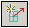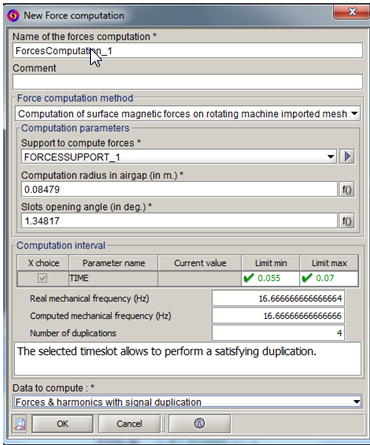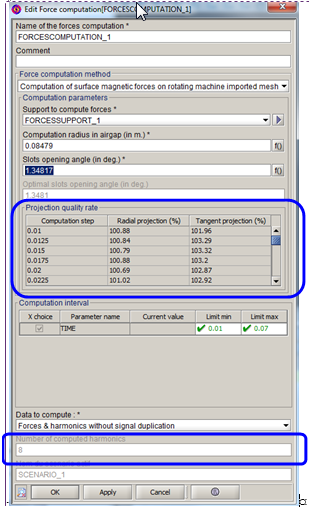# Step 2: Creation of the force computation and export of the harmonics

## Introduction

This section explains how to create a Computation of surface magnetic forces on rotating machine imported mesh.

It is available through the menu Force computation > New or through the icon## Creation of the force computation

The steps to create the force computation are described below. Images of the creation box are also available.

Step Action
1 Choose the name of the force computation (+comment)
2 Choose the method Computation of surface magnetic forces on rotating machine imported mesh
3 Choose the support created beforehand
4 Define the computation radius in airgap on ¼ distance of the airgap thickness, stator/rotor side, according to where the imported support is (see Computation radius in airgap and slots opening angle)
5 Choose the slots opening angle (See Computation radius in airgap and slots opening angle )
6

Choose the interval of computation according to the choice of applying or not a temporal duplication in the field Data to be computed (see Spatial and temporal definition).

Reminders :

• The initial step and the final step are repeated
• Do not take the first step of the scenario of the solving process as the first step of computation
7

Choose the data to be computed. You must choose :

• Application or not of a temporal duplication (choice connected to the step 6)
• The choice to calculate :
• Only the forces
• Or the forces with their harmonics

It is strongly recommended to calculate both forces and harmonics, as the calculation of the harmonics is rapid and necessary to export it

8

Verify if the time interval is in good correlation with :

• The real mechanical frequency
• The computed mechanical frequency (based on the computed interval)
• The number of duplications

(see Spatial and temporal definitionSpatial and temporal definition )

9 Validate by OK
10

Re-open the computation box and verify :

11 Visualize the arrows of the forces or of the harmonics (see Postprocessing with forces arrows )
 Step 1 to 9 :Step 10 to 12 :